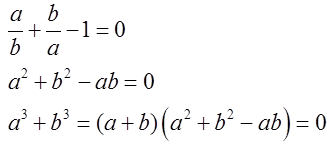# Algebra - Online Test

Q1. Simplify:Explaination / Solution: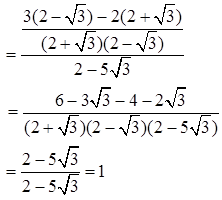Q2. Ifthen:
Explaination / Solution: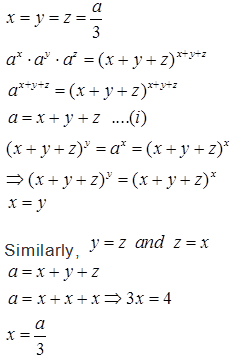Q3. Ifthen y is equal to
Explaination / Solution: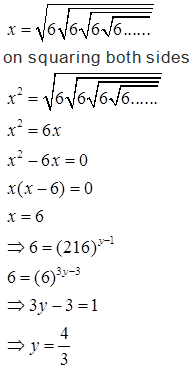Q4. Ifandthe value ofis
Explaination / Solution:

X+y=5
Cubing both sides, we get:
x3+y3+3xy(x+y)=125 OR xy = 6
((1/x) + (1/y)) = (x+y)/xy=5/6

Q5. Ifand xyz = 288, the value ofExplaination / Solution: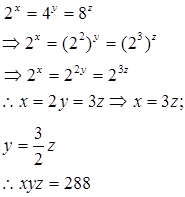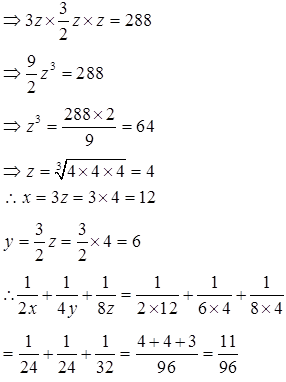Q6. If ab + bc + ca = 0, then the value ofis
Explaination / Solution:

ab + bc + ca = 0 ⇒ bc = -ab –ac and similar for others
Hence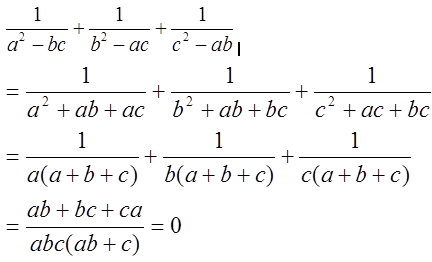Q7. Ifandthe value ofis
Explaination / Solution: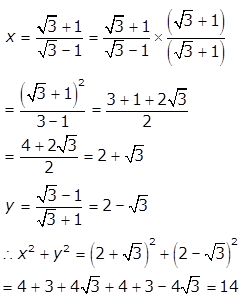Q8. Ifthen the value ofis
Explaination / Solution: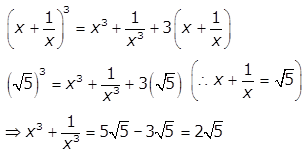Q9. If (2x)(2y) = 8 and (9x)(3y) = 81, then (x, y) is :
Explaination / Solution:

(2x)(2y) = 8 = 23
Applying the law of indices
x + y = 3----- eqn 1
(9x)(3y) = 81 = 34
2x + y = 4----- eqn 2
Solving the two equations simultaneously we get x=1
And substituting the value of x in any of the two equations y=2

Hence option A is correct

Q10. If, then the value ofis
Explaination / Solution: